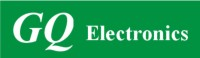Active Users: / Visits Today:
Highest Active Users:
GQ Electronics Technical Support Forum
Home | Profile | Register | Active Topics | Members | Search | FAQAll Forums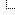GQ Electronics Forums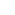3. GQ EMF EF Meter RF Spectrum Power Analyzer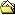How does the EMF-390 calculate the power density?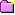New Topic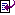Reply to Topic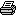Printer Friendly
Author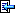Topic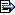dhornberger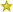2 Posts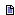Posted - 06/16/2020 :  18:52:06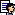I'm a Master's student doing some research that involves RF, EMF, and EF monitoring. As I've been working with the EMF-390 sensor, I've come to the point that I need to understand how the power density value that the sensor reports is calculated.According to Wikipedia here (https://en.wikipedia.org/wiki/Surface_power_density), an equation for surface power density is as follows:Pd (Watts/meter^2) = E (Volts/meter) x H (Amperes/meter)I attempted to use this equation using some values reported by the EMF sensor to determine if this was the equation you guys were using to report the power density, but I got values that didn't seem to make sense, and I'm wondering if you guys can help me understand how it's being calculated.Here is a picture of some readings obtained in Vertical Mode from which I did some calculations: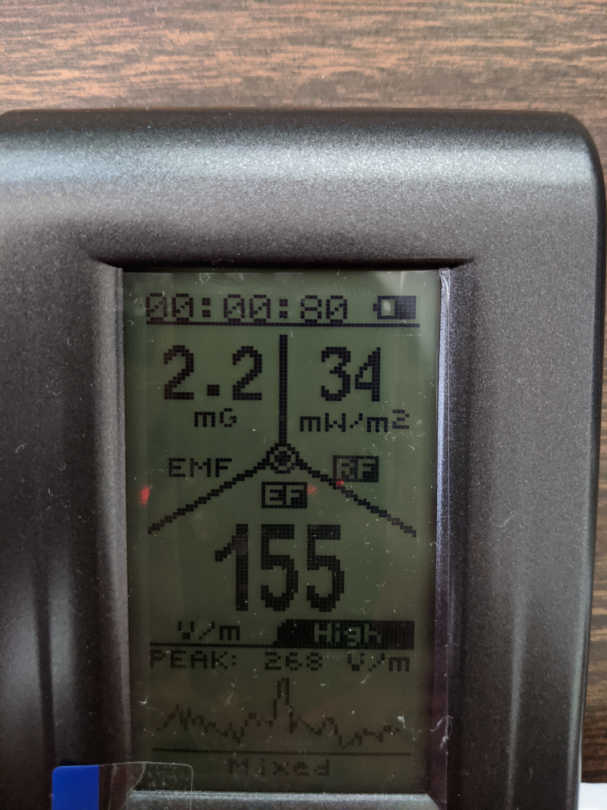As you can see, the the EMF reading is 2.2 mG, the EF reading is 155 V/m, and the RF power density is 34 mW/m^2.To use the equation I mentioned above, I converted the 2.2 mG value to Amperes/meter using this website: https://www.bussi-demagnetizers.com/en/electronic-impulse-demagnetizers/magnetic-units-converter0.0022 G = 0.175 A/mThen, I plugged the values into the equation where E = 155 V/m and H = 0.175 A/m:Pd = 155 V/m * 0.175 A/m => 27.125 W/m^2 => 27,125 mW/m^2This value of 27,125 mW/m^2 obviously doesn't equal the 34 mW/m^2 reported by the EMF sensor.Do you guys use a different equation to calculate the power density? Could you please help me be able to get at least within the same ballpark as the value reported by the sensor?Also, according to Wikipedia, I could use either of the following equations to calculate the power density since the sensor is at Far Field:Pd = E^2 � 377 OhmsPd = H^2 * 377 OhmsAgain, using the same values obtained from the sensor above, these equations render the following results respectively:Pd = (155 V/m)^2 � 377 Ohms = 63,727 mW/m^2Pd = (0.175 A/m)^2 * 377 Ohms = 11,546 mW/m^2Neither of these values are close to the power density reported by the sensor, and they aren't very close to each other either (which they should be).Any light you can shed on my confusion would be greatly appreciated.Thanks!

EmfDev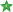1693 PostsPosted - 06/17/2020 :  09:18:06Hi dhornberger, the RF has its own sensor for RF signals. And not basing the calculation from the EMF reading or sensors.dhornberger2 PostsPosted - 06/17/2020 :  15:09:06Thank you for the quick reply!That's great to know. Thank you. Now that I know that, how does the sensor calculate the power density? If the power density being reported is independent of the EMF or EF readings, what is the power density of? Is it based off of the area of the RF sensor's antenna or a fixed distance or something? If so, what equation is being used for calculating it?Thanks again for helping me understand how this works!EmfDev1693 PostsPosted - 06/17/2020 :  15:43:57Once the RF sensor detects the power raw data of RF signal, it is converted to power density using standard calibration equipment.vfilipGreece
7 PostsPosted - 07/13/2020 :  09:43:47quote:Originally posted by dhornbergerAlso, according to Wikipedia, I could use either of the following equations to calculate the power density since the sensor is at Far Field:Pd = E^2 � 377 OhmsPd = H^2 * 377 OhmsAgain, using the same values obtained from the sensor above, these equations render the following results respectively:Pd = (155 V/m)^2 � 377 Ohms = 63,727 mW/m^2Pd = (0.175 A/m)^2 * 377 Ohms = 11,546 mW/m^2Neither of these values are close to the power density reported by the sensor, and they aren't very close to each other either (which they should be).Any light you can shed on my confusion would be greatly appreciated.Thanks!Please note that wikipedia states as : H2 = the square of the value of the magnetic field in amperes RMS squared per meter squared,E2 = the square of the value of the electric field in volts RMS squared per meter squared H end E values are the RMS values which are not measured with this device ...Posted - 08/18/2020 :  12:33:39dhornberger, My name is Ron Frazier. I am not affiliated with GQ Electronics other than as a customer. However, as my signature says, I am in training with the Building Biology Institute to be come an independent Electromagnetic Radiation Specialist (EMRS). I have been studying EMF measurement extensively and continue to do so. Always willing to learn though. I wrote an extensive forum post about the near impossibility of measuring, calculating, and quantifying RF field strength in modern digital communications systems. You may wish to look over that post cited below. For the reasons I cite in the post, every meter will read differently and there is no such thing as a universally acknowledged "correct" reading. Also, unless you're taking readings in an anechoic chamber ( https://en.wikipedia.org/wiki/Anechoic_chamber ), you will get reflections from the walls, floor, roof, and objects in the room. If you're outside, you'll get reflections from the ground, hills, mountains, buildings, cars, etc. Also, the orientation of the meter's antenna and reception pattern matters. I am under discussions with GQ here on the forum to learn more about the general RF antenna. Finally, and this is important, you CANNOT accurately measure RF signal strength at a distance less than 3 wavelengths. This is because you're in the "near field" where the EF and MF components of the RF haven't reliably merged yet. To get accurate numeric measurements, you must be in the far field. This is also discussed in the mentioned post. THAT means, if you're measuring a cell phone radiating as low as 600 MHz or .6 GHz, you have to be 5' (feet) or more away from it. If you're measuring WiFi or Bluetooth or a microwave oven at ~ 2.4 GHz, you have to be 15" (inches) away from it to get accurate numbers. Please see the post below for more info. Hope this helps.How to sweet talk your GQ EMF-390https://www.gqelectronicsllc.com/forum/topic.asp?TOPIC_ID=8856Ron -----Ron Frazier - In training with the Building Biology Institute (https://buildingbiologyinstitute.org/) to become an independent Electromagnetic Radiation Specialist (EMRS). We measure, document, analyze, and recommend mitigations for harmful EMF exposures.All my statements are mine alone though.TopicNew TopicReply to TopicPrinter Friendly Jump To: Select Forum GQ Electronics Forums       6. True USB programmer ralated topics       5. GQ Power Supply       4. GQ Hot Air Soldering Rework Station       3. GQ EMF EF Meter RF Spectrum Power Analyzer       2.GQ Geiger Muller Counter       1.Geiger Counter World Map(www.GMCmap.com)  -------------------- Home Active Topics Frequently Asked Questions Member Information Search Page
 GQ Electronics Technical Support Forum © Copyright since 2011